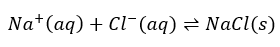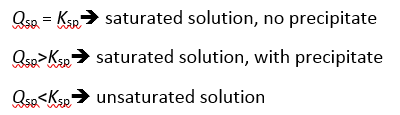# Solubility product

Ionic Equilibrium: Solubility product

The equilibrium between solids and ions is not the same as the equilibrium between gases. The equilibrium between solids and ions can be considered as a “phase” equilibrium (e.g. NaCl(aq)). Partially soluble salts form a dynamic equilibrium with the hydrated cations and anions in solution. For instance, the initial point when the solid is added to water, no ions are formed. As dissociation of salt into its ions continues, the concentration of aqueous ions increases until equilibrium is reached. At equilibrium, the rate of the dissolution process is equal to the rate of precipitation. This process can be represented by the solubility product constant or Ksp expression.

Consider common salt dissolving in water:Figure : Precipitation of salt in water.

Solubility is the quantity of a substance that dissolves to form a saturated solution.Molar solubility is the number of moles of the solute that dissolves to form a litre of saturated solution. An equilibrium is established when the amount dissolving equals the amount precipitating. This can only be true if there is some solid. A solution with solid remaining (I.e. in equilibrium) is called “saturated”.The solubility product, Ksp is the equilibrium constant for the equilibrium between an ionic solid and its saturated solution.

Solubility Product (Ksp) = [products]x/[reactants]y

When reactants are in the solid state, Ksp=[products]x

AaBb(s) aAb+ (aq) + bBa- (aq)

Ksp = [Ab+]a [Ba-]b

A2B3(s) 2A3+ + 3B2–;Ksp=[A3+]2 [B2–]3

For example:

Ca(IO3)2(s) ⇌ Ca2+(aq) + 2 IO3(aq); Ksp = [Ca2+][IO3]2 = 7.1 x 10-7

If the solubility of Ca(IO3)2(s) is s mol/L, then:

Ksp = 4s3 = 7.1 x 10-7

s = 5.6 x 10-3 mol/L

Certain factors affect the solubility product. Solubility generally increases with temperature. Common ions reduce solubility while the formation of a complex ion increases solubility. Complex ions are ions consisting central metal ions and ligands covalently bonded to the metal ions; Ligands can be neutral molecules such as H2O, CO, and NH3, or anions such as Cl, F, OH, and CN. The salt effect also increases solubility. Likewise, pH affects the solubility of ionic compounds in which the anions are conjugate bases of weak acids.For example, the solubility of compounds such as Ag3PO4, with anions that are conjugate bases of weak acids, increases as the pH is lowered by adding nitric acid.The solubility of compounds of the type M(OH)n decreases as pH is increased and increases as pH are decreased.

To predict the formation of precipitation we should consider the relationship between Qsp and Ksp.Qsp is ion product expressed in the same way as Ksp for a system.Compounds with different solubility can be selectively precipitated by adjusting the concentration of the precipitating reagents.For example, in a solution containing two salts such as AgCl and PbCl2, AgCl has a much lower Ksp than PbCl2. If Ag+ and Pb2+ are present in the same solution, the Ag+ ion can be selectively precipitated as AgCl, leaving Pb2+ in solution.Figure : Separating the common cations by selective precipitation.